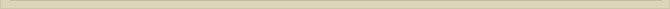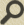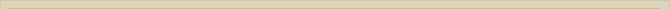## The EM-rotator

When you are interested in physics you must read “Unbelievable“!

When both masses are not connected through a rigid mass less rod and are charged masses, like the electron (Me) and proton (Mp) in the hydrogen atom, the above properties of the mechanical free rotator must also be valid in stable situations.

To obtain a free EM-rotator with stable orbits, the function of the mass less rod must be taken over forces working on electrons and nucleus in the atom. All forces must be neutralized/equalized during the rotation of the electron around the proton at any time.

In a stable situation, the following mechanical conditions for proton (Mp) and electron (Me) in the hydrogen atom must be valid: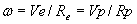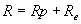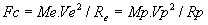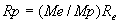The centrifugal force Fc, working equally on proton and electron, must be compensated internal forces; the electrostatic force Fe. We consider the EM-free rotator where the centrifugal force (Fc) is compensated at all times  the electrostatic force (Fe). This assumption implicitly assumes that the electron is in a steady orbit around the proton.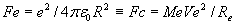and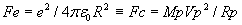The equilibrium solution for the free and stable EM-rotator is: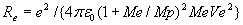(1)

Where Re is the distance of the electron to the rotation point of the system (figure 1), Me the mass of the electron, Mp the mass of the proton, Ve the rotation speed of the electron, e the elementary charge of the electron and εo the dielectric constant in vacuum.

Remark: In the previous chapters the elementary charge of the electron was named “Qe”. In this chapter the elementary charge of the electron is called “e” because in physics articles that is the normally used symbol for the charge of an electron.

This equation describes the radius Re of the orbiting electron as a function of the rotation speed Ve in the situation where the system is stable (dE/dt=0) and the orbiting speed is constant (dVe/dt=0). Analyzing this equation we observe that for any speed of the electron Ve there is a possible solution Re.

The situation in figure 1 sketches also the situation where the electron and proton are both in a steady orbit around O. The electrostatic force Fe compensates now the centrifugal force Fc.

As there is for any speed of the electron Ve also an orbit distance Re where all forces are in equilibrium, there are infinite solutions and so there are no theoretical solutions based on this model that resemble the reality of fixed energy levels.

The proton and electron have, besides mass, also a charge. Because proton and electron do not move relatively to each other in a stable orbiting situation there are no induced magnetic fields.

The moving electron and proton would each present an electric current when the centrifugal force is not (completely) compensated the electrostatic force. The magnetic fields induced electron and nucleus, when there is no electrostatic force (Fe=0), would induce a maximum magnetic force Fm between proton and electron equal to: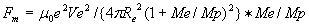This magnetic force Fm, when relevant, would be negligible compared to the electrostatic force Fe. The nuclear forces  are negligible at the molecular distance.

A moving charge presents dynamic energy in the form of magnetic energy. The Rutherford-model was considered not stable because the circling electrons around the nucleus would loose energy emitting radiation; so a stable EM-rotator like the Rutherford-model was considered impossible. A moving charge can emit radiation and loose energy, but it is not true that a moving charge in all circumstances has to loose energy. We refer to the article “The Equivalence of magnetic and Kinetic Energy” where it is proven that both energy forms are identical. A moving mass can infinite circle without loosing energy and so can a charge. When there is abundance of energy a charge may oscillate. This oscillation energy is redundant energy and can therefore induce/emit electromagnetic radiation.

Next chapter: The Energy Level of the Hydrogen EM-free Rotator Atom